Next: Incompressible Viscous Flow Up: Incompressible Aerodynamics Previous: Simple Flight Problems

# Exercises

1. Consider the integral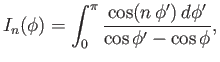whereis a non-negative integer. This integral is defined by its Cauchy principal value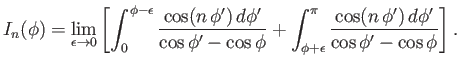As was demonstrated in Section 9.9,Show thatand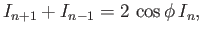and, hence, that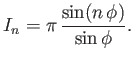2. Suppose that an airfoil of negligible thickness, and wingspan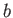, has a width whosevariation is expressed parametrically asfor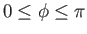, whereShow that the air circulation about the airfoil takes the form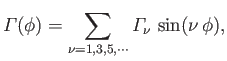where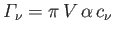. Here,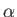is the angle of attack (which is assumed to be small). Demonstrate that the downwash velocity at the trailing edge of the airfoil is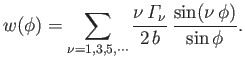Hence, show that the lift and induced drag acting on the airfoil take the values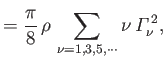respectively. Demonstrate that the drag to lift ratio can be writtenwhereis the aspect ratio. Hence, deduce that the airfoil shape (in the-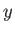) plane that minimizes this ratio (at fixed aspect ratio) is an ellipse (i.e., such thatfor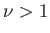).

3. Consider a plane that flies with a constant angle of attack, and whose thrust is adjusted such that it cancels the induced drag. The plane is effectively subject to two forces. First, its weight,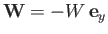, and second its lift. Here,andare horizontal and vertical coordinates, respectively,is the plane's instantaneous velocity, and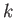is a positive constant. Note that the lift is directed at right angles to the plane's instantaneous direction of motion, and has a magnitude proportional to the square of its airspeed. Demonstrate that the plane's equations of motion can be written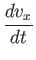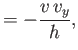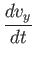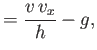whereis a positive constant with the dimensions of length. Show that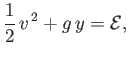whereis a constant. Suppose that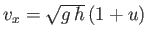and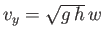, where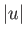,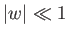. Demonstrate that, to first order in perturbed quantities,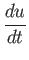Hence, deduce that if the plane is flying horizontally at some speed, and is subject to a small perturbation, then its altitude oscillates sinusoidally at the angular frequency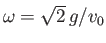. This type of oscillation is known as a phugoid oscillation.Next: Incompressible Viscous Flow Up: Incompressible Aerodynamics Previous: Simple Flight Problems
Richard Fitzpatrick 2016-03-31